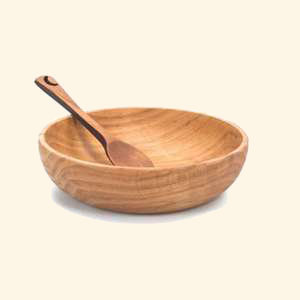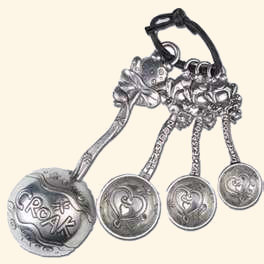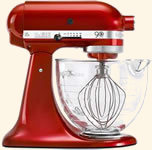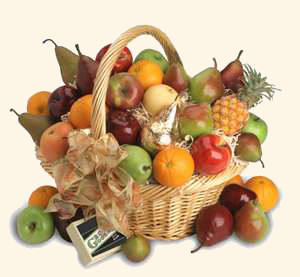Cooking ConversionsPounds to Kilograms Conversions (lb to kg)

When embarking on larger projects such as wedding cakes, jam making or wine making you will find that instead of ounces or grams, you will see pounds or kilograms in your recipe. The conversion for pounds to kilograms and kilograms to pounds are given below.

Pounds to Kilograms Converter

Type a value in either field to convert from pounds to kilograms (lb to kg) or kilograms to pounds (kg to lb) (results are accurate to three decimal places).

 Pounds Kilograms lb = kg

Common Pound to Kilogram Conversion Table

Most of the common pound to kilogram conversions are given in the table below (results are rounded to 3 decimal places - the nearest gram).

 Pounds 1 2 3 4 5 6 7 8 9 10 Kilograms 0.454 0.907 1.361 1.814 2.268 2.722 3.175 3.629 4.082 4.536

Full Pound to Kilogram Conversion Tables

Pound to Kilogram Conversion Formula

The conversion formula for pounds to kilograms (lb to kg) is 1 pound = 0.45359237 kilograms.

Common Kilogram to Pound Conversion Table

Commonly used values for kilogram to pound conversions used in cooking are given in the table below (results are rounded to one decimal place).

 Kilograms 0.5 1 1.5 2 3 4 5 10 20 Pounds 1.1 2.2 3.3 4.4 6.6 8.8 11 22 44.1

Kilogram to Pound Conversion Formula

The conversion formula for kilograms to pounds (kg to lb) is 1 kilogram = 2.20462262 pounds.

Other weight conversions for cooking